# Calculate Equivalent Circulation Density (ECD) with complex engineering equations

These formulas below are used for complex calculation for annular pressure loss and equivalent circulating density. I think this calculation will give you more accurate result than a simple equation. Please follow the following steps how to calculate annular pressure loss and ECD.

1. Determine n:2. Determine K: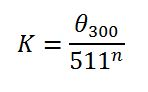3. Determine annular velocity (v) in ft/min:4. Determine critical velocity (Vc) in ft/min: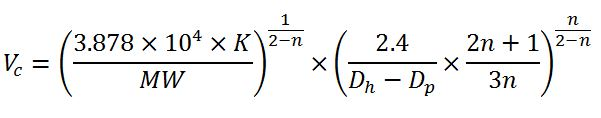5. Pressure loss for laminar flow (Ps), psi:6. Pressure loss for turbulent flow (Ps), psi: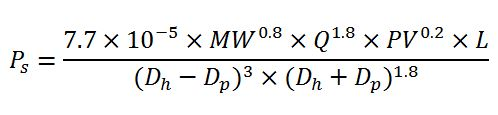7. Determine equivalent circulating density (ECD), ppg:Abbreviation meaning

θ300: viscometer dial reading at 300 rpm
θ600: viscometer dial reading at 600 rpm
Q: Flow rate in gpm
Dh: Diameter of hole
Dp: Diameter of drill pipe, drill collar or BHA in ft
v: annular velocity in ft/min
L: length of drill pipe, drill collar or BHA in ft
MW: Mud Weight
PV: Plastic viscosity

Example: Equivalent circulating density (ECD) in ppg by using following data:

Mud weight = 9.5 ppg
θ300 = 40
θ600 = 60
Plastic viscosity = 20 cps
Circulation rate = 650 gpm
Hole diameter = 8.5 in.
Drill collar OD = 6.75 in.
Drill pipe OD = 5.0 in
Drill collar length = 600 ft
Drill pipe length = 10,000 ft
True vertical depth = 9,000 ft

1. Determine n: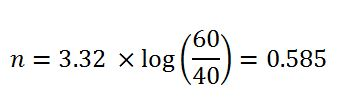2. Determine K: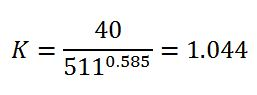3. Determine annular velocity (v) in ft/min around drill pipe: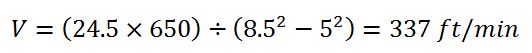4. Determine critical velocity (Vc) in ft/min around drill pipe: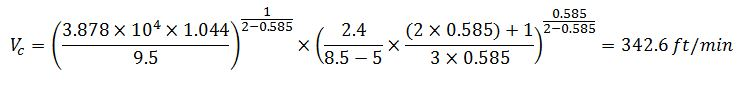The annular velocity around drill pipe is less than the critical velocity around drill pipe so this is laminar flow. The equation #5 (for laminar flow) must be applied in this case.

Pressure loss for turbulent flow (Ps), psi:5. Determine annular velocity (v) in ft/min around drill collar: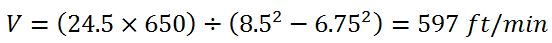6. Determine critical velocity (Vc) in ft/min around drill collar: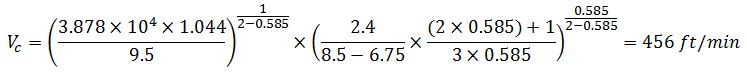The annular velocity around drill collar is more than the critical velocity around drill collar so this is turbulent flow. The equation #6 (for turbulent flow) must be applied in this case.

Pressure loss for laminar flow (Ps), psi: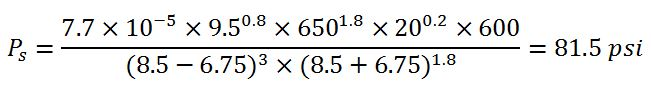Total annular pressure loss = annular pressure loss around drill pipe + annular pressure loss around drill collar

Ps=271.3+81.5 = 352.8psi

7. Determine equivalent circulating density (ECD), ppg:Please find the Excel sheet for calculating ECD (engineering calculation)

Ref books: Lapeyrouse, N.J., 2002. Formulas and calculations for drilling, production and workover, Boston: Gulf Professional publishing.

Bourgoyne, A.J.T., Chenevert , M.E. & Millheim, K.K., 1986. SPE Textbook Series, Volume 2: Applied Drilling Engineering, Society of Petroleum Engineers.

Mitchell, R.F., Miska, S. & Aadny, B.S., 2011. Fundamentals of drilling engineering, Richardson, TX: Society of Petroleum Engineers.

### Related Post

Amount of cuttings produced per foot of hole and t... After learning about capacity calculation, we can apply the capacity calculation to determine how much barrels of cutting produced per foot of hole dr...
Lost Circulation and Well Control Lost circulation is a situation when drilling fluid losses downhole because formation(s) is fractured. There are three levels of lost circulation whic...
System Constrain for Drilling Hydraulics When we get the optimum flow rate which match your optimization objective, you must check the system constrains such as • Deliverability of mud pum...
Kick Tolerance Calculation This post will teach you how to calculate kick tolerance. This concept is very important to well design and will give you an idea how much kick you ca...
Share the joy
• 9
•
•
•
•
•
•
•
•
9
SharesWorking in the oil field and loving to share knowledge.

### 10 Responses to Calculate Equivalent Circulation Density (ECD) with complex engineering equations

1.Lewis Hase says:

Excellent Blog for those interested in drilling. Congratulations.Very educational.
Suggestion: A few pictures of equipment or screens would be very
useful.
Lewis Hase.

2.Mufti Chevalier says:

Thanks for share this calculation along with the example, really help me

3.Imtiaz Ahmed says:

Very much helpful for calculating the other related stuffs

4.Mohammed says:

Hello

just wanted to let you know that all the answers are correct, but i think there is a typo in the formula…. in the determination of (K), you should actually use the 300 rpm reading instead of the 600 rpm reading… the execution of the formula is OK, its just the way it was written.

Mohammed

•DrillingFormulas.Com says:

The calculation uses 600 reading for calculation.

5.Mike says:

There is a problem with your data. With the readings you give from your Viscometer – your YP is 0. You report PV -= 22 cp. THe PV is actually 30 cP. THere are other problems as well.

•DrillingFormulas.Com says:

Mike,

All information and calculation has been updated.

Regards,
Shyne

6.Muahmmad Ali says:

0.052 is the constant and it is different in onshore and off shore… can you tell me how it is calculated.

•DrillingFormulas.Com says:

0.052 can be used for any location (onshore or offshore).

7.Princeton University says:

It’s surprising to find on drillingformulas.com a
resource so precious about equations.
We will note your page as a benchmark for Calculate Equivalent Circulation Density (ECD) with
complex engineering equations‎.
We also invite you to link and other web resources for equations like http://equation-solver.org/ or https://en.wikipedia.org/wiki/Equation.
Thank you ang good luck!

This site uses Akismet to reduce spam. Learn how your comment data is processed.